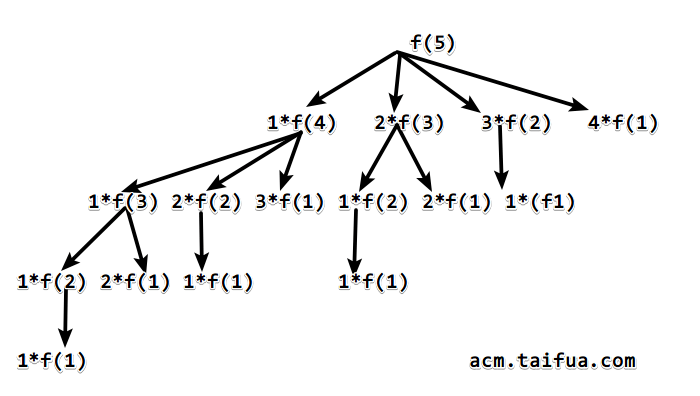# LeetCode343. Integer Break（动态规划）

## 2019 年 03 月 24 日 • 阅读: 1912 • LeetCode • 阅读设置

### LeetCode343. Integer Break（动态规划）

• Medium
• Accepted：75,299
• Submissions：158,763

Given a positive integer n, break it into the sum of at least two positive integers and maximize the product of those integers. Return the maximum product you can get.

Example 1:

Input: 2
Output: 1
Explanation: 2 = 1 + 1, 1 × 1 = 1.

Example 2:

Input: 10
Output: 36
Explanation: 10 = 3 + 3 + 4, 3 × 3 × 4 = 36.

Note: You may assume that n is not less than 2 and not larger than 58.

### 链接

https://leetcode.com/problems/integer-break

### 题解### 代码

• Runtime: 4 ms, faster than 100.00% of C++ online submissions for Integer Break.
• Memory Usage: 8.5 MB, less than 19.32% of C++ online submissions for Integer Break.
class Solution {
private:
vector <int> vis;
int solve(int n)
{
if (n == 1)
return 1;
if (vis[n] != -1)
return vis[n];
int res = -1;
for (int i = 1; i < n; ++i)
res = max(max(res, i * (n - i)), i * solve(n - i));
return vis[n] = res;
}
public:
int integerBreak(int n) {
vis = vector<int>(n + 1, -1);
return solve(n);
}
};
class Solution {
public:
int integerBreak(int n) {
vector <int> ans(n + 1, 1);
for (int i = 2; i <= n; ++i)
for (int j = 1; j < i; ++j)
ans[i] = max(max(ans[i], j * (i - j)), j * ans[i - j]);
return ans[n];
}
};

The end.
2019年3月24日 星期日

### 参考

The first thing we should consider is : What is the max product if we break a number N into two factors?

I use a function to express this product: f=x(N-x)

When x=N/2, we get the maximum of this function.

However, factors should be integers. Thus the maximum is (N/2)(N/2) when N is even or (N-1)/2 (N+1)/2 when N is odd.

When the maximum of f is larger than N, we should do the break.

(N/2)*(N/2)>=N, then N>=4

(N-1)/2 *(N+1)/2>=N, then N>=5

These two expressions mean that factors should be less than 4, otherwise we can do the break and get a better product. The factors in last result should be 1, 2 or 3. Obviously, 1 should be abandoned. Thus, the factors of the perfect product should be 2 or 3.

The reason why we should use 3 as many as possible is

For 6, 3 3>2 2 * 2. Thus, the optimal product should contain no more than three 2.

Below is my accepted, O(N) solution.

public class Solution {
public int integerBreak(int n) {
if(n==2) return 1;
if(n==3) return 2;
int product = 1;
while(n>4){
product*=3;
n-=3;
}
product*=n;

return product;
}
}

I saw many solutions were referring to factors of 2 and 3. But why these two magic numbers? Why other factors do not work?
Let's study the math behind it.

For convenience, say n is sufficiently large and can be broken into any smaller real positive numbers. We now try to calculate which real number generates the largest product.
Assume we break n into (n / x) x's, then the product will be xn/x, and we want to maximize it.

Taking its derivative gives us n * xn/x-2 * (1 - ln(x)).
The derivative is positive when 0 < x < e, and equal to 0 when x = e, then becomes negative when x > e,
which indicates that the product increases as x increases, then reaches its maximum when x = e, then starts dropping.

This reveals the fact that if n is sufficiently large and we are allowed to break n into real numbers,
the best idea is to break it into nearly all e's.
On the other hand, if n is sufficiently large and we can only break n into integers, we should choose integers that are closer to e.
The only potential candidates are 2 and 3 since 2 < e < 3, but we will generally prefer 3 to 2. Why?

Of course, one can prove it based on the formula above, but there is a more natural way shown as follows.

6 = 2 + 2 + 2 = 3 + 3. But 2 * 2 * 2 < 3 * 3.
Therefore, if there are three 2's in the decomposition, we can replace them by two 3's to gain a larger product.

All the analysis above assumes n is significantly large. When n is small (say n <= 10), it may contain flaws.
For instance, when n = 4, we have 2 * 2 > 3 * 1.
To fix it, we keep breaking n into 3's until n gets smaller than 10, then solve the problem by brute-force.